We learn about fractions in elementary school math. When we calculate fractions, we use multiplication. Multiplication of fractions is easier than addition and subtraction. This is because we don’t need to make the denominators the same by finding the common denominator.

However, when multiplying, we need to simplify the fractions. Also, when multiplying fractions, it is necessary to use improper fractions instead of mixed fractions.

In addition, if you can simplify fractions, it is common to simplify fractions before multiplying. This is because it reduces miscalculations.

Although it is easier than addition and subtraction, there is a correct way to multiply fractions, and you have to learn how to do it. So, let’s take a look at how to multiply fractions.

## How to Multiply Fractions by Fractions

First, let’s learn how to multiply fractions. When multiplying fractions, the process is simple. Let’s multiply the denominators and numerators, respectively. It looks like this.

• $\displaystyle\frac{3}{4}×\displaystyle\frac{5}{2}=\displaystyle\frac{3×5}{4×2}=\displaystyle\frac{15}{8}$

In this way, we multiply the denominators by each other and the numerators by each other. The denominators do not have to be the same number, unlike in addition and subtraction. Therefore, unlike addition and subtraction, we do not have to find a common denominator when multiplying fractions.

The multiplication of fractions is not particularly difficult, as you only need to multiply the denominators by each other and the numerators by each other.

### Multiplication of Integers by Fractions: Converting Integers to Fractions

So how do we multiply integers by fractions? For example, calculate the following expression.

• $2×\displaystyle\frac{2}{3}$

To understand this calculation, let’s turn 2 into a fraction: if we turn 2 into a fraction, we can use $\displaystyle\frac{2}{1}$. Integers are also fractions. However, if the denominator is 1, we usually omit the denominator. Therefore, it is important to understand that although the integer is written as 2, it can also be written as $\displaystyle\frac{2}{1}$.

By changing 2 to $\displaystyle\frac{2}{1}$, we can multiply fractions. It will look like this.

• $2×\displaystyle\frac{2}{3}=\displaystyle\frac{2}{1}×\displaystyle\frac{2}{3}=\displaystyle\frac{4}{3}$

It is difficult to understand how to calculate with integers. So if we change the integers to fractions, we will be able to understand how to do the calculations.

### Why Can We Multiply Numerators by Each Other and Denominators by Each Other?

So why is it possible to multiply fractions without making the denominators the same? To understand this, let’s learn about the properties of multiplication.

Multiplying fractions is the same as dividing and multiplying at the same time. Fractions can be converted to division as follows.

• $\displaystyle\frac{4}{9}=4÷9$
• $\displaystyle\frac{13}{2}=13÷2$

When we divide a number, the denominator will be larger. The denominator is what divides the number into equal parts. For example, $\displaystyle\frac{1}{2}$ means to divide the number into two equal parts. Also, $\displaystyle\frac{1}{3}$ means to divide the number into three equal parts.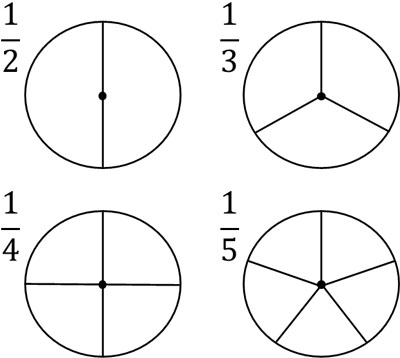Therefore, when multiplying fractions, multiplying the denominators by each other will result in a larger number in the denominator. For example, $\displaystyle\frac{1}{2}×\displaystyle\frac{1}{3}$ results in $\displaystyle\frac{1}{6}$ by multiplying the denominators.

Also, $\displaystyle\frac{1}{2}×\displaystyle\frac{1}{3}$ means to divide $\displaystyle\frac{1}{2}$ into three equal parts. In the figure, it looks like this.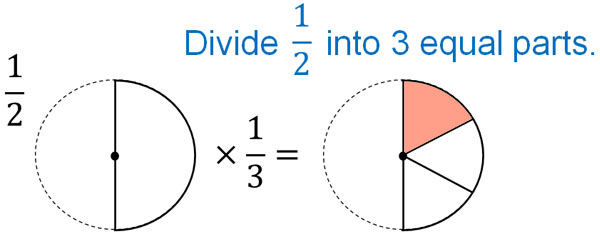The denominator represents the number to be divided, and when multiplying fractions, the denominators are multiplied together.

The numerator represents the number of fractions. For example, $\displaystyle\frac{2}{5}$ is the sum of two $\displaystyle\frac{1}{5}$. Also, $\displaystyle\frac{3}{5}$ is the sum of three $\displaystyle\frac{1}{5}$.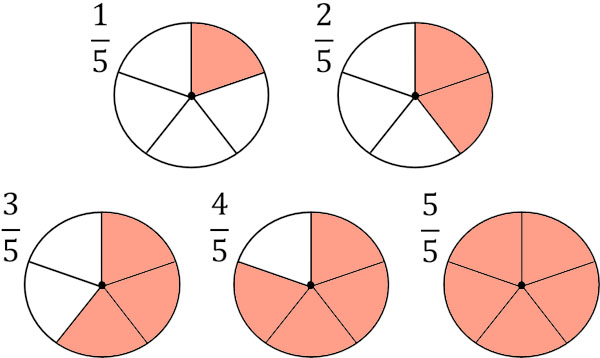In other words, the numerator is how many we need to collect after dividing.

So how do we think of $\displaystyle\frac{1}{2}×\displaystyle\frac{3}{4}$? In order to multiply $\displaystyle\frac{3}{4}$, let’s divide $\displaystyle\frac{1}{2}$ into four equal parts and then collect the three. The result is as follows.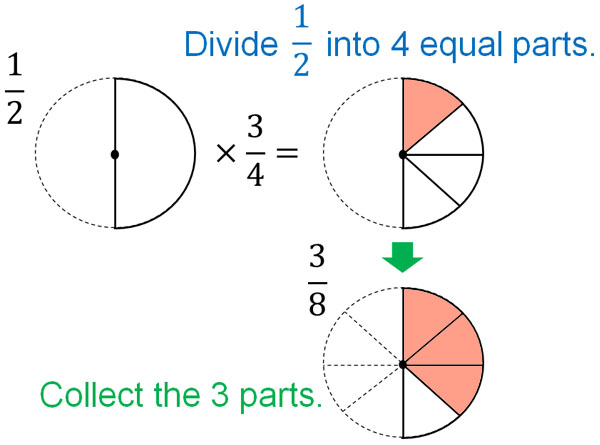If you check the figure, you will see that $\displaystyle\frac{1}{2}$ is divided into 4 equal parts, so the denominator is 8. In this way, when multiplying fractions, the denominators are multiplied together.

Also, the numerator of $\displaystyle\frac{3}{4}$ is 3. Therefore, we need to collect three after dividing. In other words, when multiplying $\displaystyle\frac{3}{4}$, we divide by 4 and then multiply by 3. This means that we are multiplying the numerators by each other.

Therefore, the calculation is as follows.

• $\displaystyle\frac{1}{2}×\displaystyle\frac{3}{4}＝\displaystyle\frac{1×3}{2×4}＝\displaystyle\frac{3}{8}$

As you can see, when multiplying fractions, the answer is obtained by multiplying the numerators by each other and the denominators by each other.

## Change Mixed Fractions to Improper Fractions When Multiplying Fractions

How do we calculate the multiplication of mixed fractions? When adding or subtracting mixed fractions, you can calculate them as mixed fractions. For example, you can calculate as follows.

• $1\displaystyle\frac{1}{4}+2\displaystyle\frac{2}{4}=3\displaystyle\frac{3}{4}$

On the other hand, do not multiply using mixed fractions. If you multiply with mixed fractions, you will get the wrong answer. For example, how do we calculate the following multiplication?

• $1\displaystyle\frac{1}{4}×1\displaystyle\frac{1}{2}$

As with addition, if you separate the integer from the fraction, you’ll make a mistake. If you check the integer parts of the two mixed fractions, they are both 1. Therefore, $1×1=1$. Also, if we multiply the two fractions together, we get $\displaystyle\frac{1}{4}×\displaystyle\frac{1}{2}=\displaystyle\frac{1}{8}$. Therefore, many people think that $1\displaystyle\frac{1}{4}×1\displaystyle\frac{1}{2}=1\displaystyle\frac{1}{8}$ is the answer.

However, this answer is wrong. $1\displaystyle\frac{1}{2}$ is a number greater than 1. Therefore, when we multiply by $1\displaystyle\frac{1}{2}$, the answer must be greater than $1\displaystyle\frac{1}{4}$. The previous answer, $1\displaystyle\frac{1}{8}$, is a smaller number than $1\displaystyle\frac{1}{4}$. Therefore, we can see that it is obviously the wrong answer.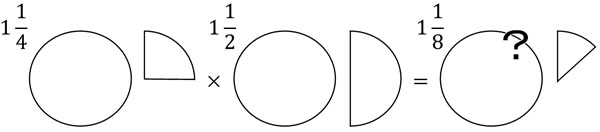In any case, it is important to understand that multiplication with mixed fractions will result in wrong answers.

So, how can we multiply with mixed fractions? Let’s consider the following calculation.

• $\displaystyle\frac{1}{4}×1\displaystyle\frac{1}{2}$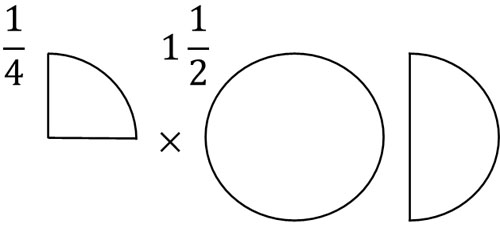$1\displaystyle\frac{1}{2}=1+\displaystyle\frac{1}{2}$. In other words, $\displaystyle\frac{1}{4}×1\displaystyle\frac{1}{2}$ means that there is one $\displaystyle\frac{1}{4}$ and half of $\displaystyle\frac{1}{4}$. Therefore, we have the following.

$\displaystyle\frac{1}{4}×1\displaystyle\frac{1}{2}$

$=\displaystyle\frac{1}{4}×1+\displaystyle\frac{1}{4}×\displaystyle\frac{1}{2}$

$=\displaystyle\frac{1}{4}+\displaystyle\frac{1}{8}$

$=\displaystyle\frac{2}{8}+\displaystyle\frac{1}{8}$

$=\displaystyle\frac{3}{8}$

Thus, we can get the answer.

However, it is complicated to do the calculation with the mixed fractions. So, let’s change the mixed fractions to improper fractions. This will make the calculation easier, as shown below.

• $\displaystyle\frac{1}{4}×1\displaystyle\frac{1}{2}=\displaystyle\frac{1}{4}×\textcolor{red}{\displaystyle\frac{3}{2}}=\displaystyle\frac{3}{8}$

If it is an improper fraction (or a proper fraction), you can multiply the numerators by each other and the denominators by each other. However, in a multiplication of mixed fractions, you should change them to improper fractions.

## Multiplying Fractions When Fractions Can Be Simplified

So far, we have discussed multiplication when fractions are not simplified. On the other hand, if you can simplify fractions, you need to simplify fractions when multiplying.

Even if the numerator and denominator are divided by the same number, the answer will be the same. So, if you can simplify fractions, make sure to simplify fractions. It looks like this.

• $\displaystyle\frac{2}{3}×\displaystyle\frac{6}{3}=\displaystyle\frac{12}{9}=\displaystyle\frac{4}{3}$

If you do not simplify the fractions, you will get the wrong answer. Therefore, we need to find the common factors of the numerator and denominator and simplify the fractions.

### Simplify Fractions Before Multiplying to Make Numbers Smaller

When multiplying, make sure to simplify the fractions first. In other words, simplify the fractions before multiplying rather than after answering.

If you do the multiplication first, the calculation will look like this.

• $\displaystyle\frac{3}{7}×\displaystyle\frac{14}{5}=\displaystyle\frac{42}{35}=\displaystyle\frac{6}{5}$

The greatest common divisor for 42 and 35 is 7. So we divide the numerator and denominator by 7 to get the answer. However, it is hard to find the common factor of large numbers. So, before we multiply, let’s simplify fractions.

Simplifying fractions is the same as doing division. Also, when using the same number, the answer will be the same if you divide after multiplication or if you multiply after division. For example, the following will give the same answer.

• $6\textcolor{red}{÷2×4}=12$
• $6\textcolor{red}{×4÷2}=12$

When doing calculations, we usually calculate in order from left to right. As a result, although the order is different for $÷2×4$ and $×4÷2$, the answer will be the same. This is the same for fractions, so instead of doing division after multiplication, we should do multiplication after division.

For $\displaystyle\frac{3}{7}×\displaystyle\frac{14}{5}$, let’s check the numerator and denominator. In simplifying fractions, the numerator and denominator are divided by the same number. So, check the numerator and denominator, and if you can divide by the same number, do the division first. The result is as follows.If you look at 14 in the numerator and 7 in the denominator, the common divisor is 7. So let’s divide both numbers by 7. Then you can change $\displaystyle\frac{3×14}{7×5}$ to $\displaystyle\frac{3×2}{1×5}$. As a result, you can multiply fractions easily.

When multiplying fractions, make sure to do the division before multiplying. This will reduce the number of miscalculations and will also allow you to calculate quickly.

## Multiplication Methods and Simplifying Fractions are Important

Compared to addition and subtraction, multiplication of fractions is much easier. You don’t need to find the least common denominator; just multiply the numerators by each other and the denominators by each other. It is important to understand the multiplication of fractions not only when multiplying fractions by fractions, but also when multiplying integers by fractions.

Also, when using mixed fractions, do not multiply. Always change them to improper fractions. If you want to multiply, it must be with improper fractions (or proper fractions).

In addition to that, simplify fractions. Rather than simplifying fractions after multiplication, you can simplify fractions before multiplication. This way, you can prevent the numbers from getting bigger and avoid miscalculations.

The important points in multiplying fractions are how to calculate multiplication and simplify fractions. Let’s use these two things to multiply fractions.